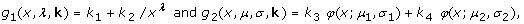Volume 10 Supplement 1

# Predicting BCI performance to study BCI illiteracy

## Introduction and method

Brain-Computer Interfaces (BCIs) allow a user to control a computer application by brain activity as acquired by EEG, for example. One of the biggest challenges in BCI research is to understand and antagonize the problem of "BCI Illiteracy:" BCI control does not work for a non-negligible portion of subjects (estimated 15% to 30%). To shed light on this problem, we developed a neurophysiological predictor of BCI performance, which can be determined from a two minutes recording of a "relax with eyes open" condition using three Laplacian channels. In a screening study, N = 40 subjects performed first a calibration measurement of kinesthetic motor imagery with the left hand, right hand and both feet. A classifier was trained on the modulation of sensorimotor rhythms (SMRs) for the pair of classes that provided best discrimination similar to . In a subsequent feedback measurement subjects could control a 1D cursor application using that classifier. Feedback accuracy largely varied between subjects. Here we present a predictor that quantifies the strength of the SMR idle rhythm during rest. To determine the value of the proposed SMR predictor, a short (~two minutes) recording under the condition "relax with eyes open" using three Laplacian channels at C3, Cz, C4 is required. In each channel the power spectral density (PSD) is calculated and the maximum difference between the PSD curve and a fit of the 1/f noise spectrum is calculated as follows. Denoting the probability density function of a normal distribution with mean μ and standard deviation σ by φ(·; μ, σ), the PSD is modeled by a function g(x, λ, μ, σ, k) = g1 (x, λ, k)+ g2 (x, μ, σ, k) withwhere k i , λ R. Function g1 models the noise spectrum and g2 models two peaks in the PSD, which are typically at the alpha and beta frequency. After the parameters have been found by a standard optimization procedure to minimize the mean squared error between g and the estimated PSD, the value of the SMR predictor is the average across the three channels of the maximum of g2 that is found either in the vicinity of μ1 or μ2: see Figure 1.

## Result and discussion

Interestingly, this SMR predictor explains as much as R2 = 37% of the variance in feedback accuracy in our sample. This finding not only points out a single cause as essential property for successful performance with an SMR-based BCI, but thereby also paves a way to approach the BCI illiteracy problem. Further studies will evaluate a specifically tailored neurofeedback training in order to enhance the SMR idle rhythm and, as may be speculated, feedback performance in subsequent BCI applications.

## References

1. Blankertz B, Tomioka R, Lemm S, Kawanabe M, Müller KR: Optimizing spatial filters for robust EEG single-trial analysis. IEEE Signal Proc Magazine. 2008, 25: 41-56. 10.1109/MSP.2008.4408441.

## Acknowledgements

This work was supported in part by grants of the DFG (MU 987/3-1) and the BMBF (01IB001A/B, 01GQ0850).

## Author information

Authors

### Corresponding author

Correspondence to Benjamin Blankertz.

## Rights and permissions

Reprints and Permissions